# Adding sociology and psychology panes to VisibLie_E8 MyToE Demonstration

These are more personal interest than hard math/science as the other panes – but they are, IMO, interesting asides.

The sociology pane deals with the idea of Old Testament Hebrew and New Testament Koine Greek Gematria. The histogram shows the distribution of words used in the Bible according to their gematria value in Hebrew or Greek. Each word in each verse is selectable and shown in the distribution. Each word in the verse is colored by their value in Hebrew (if Old Testament), Greek and English.
Note: Hebrew is properly presented from right to left.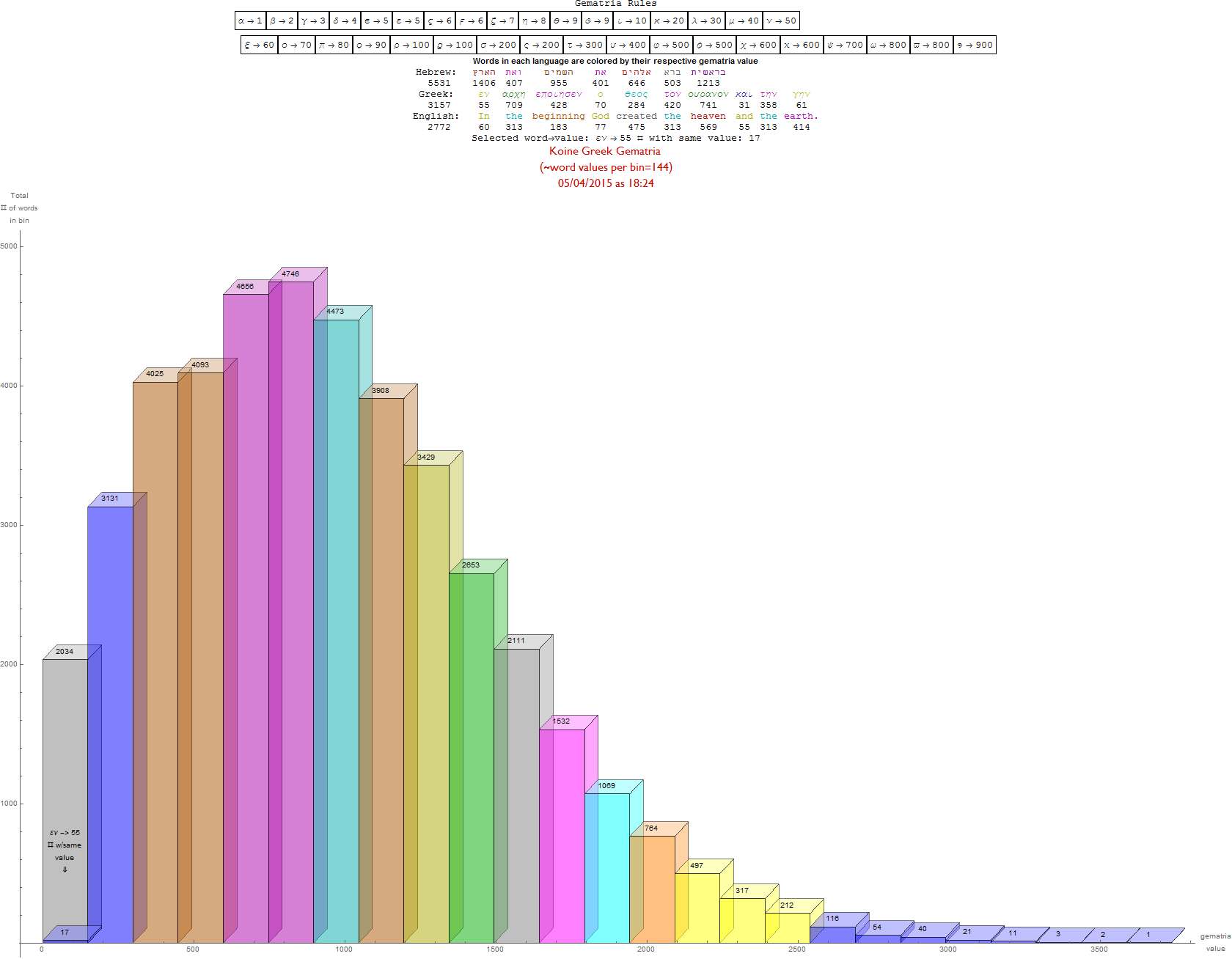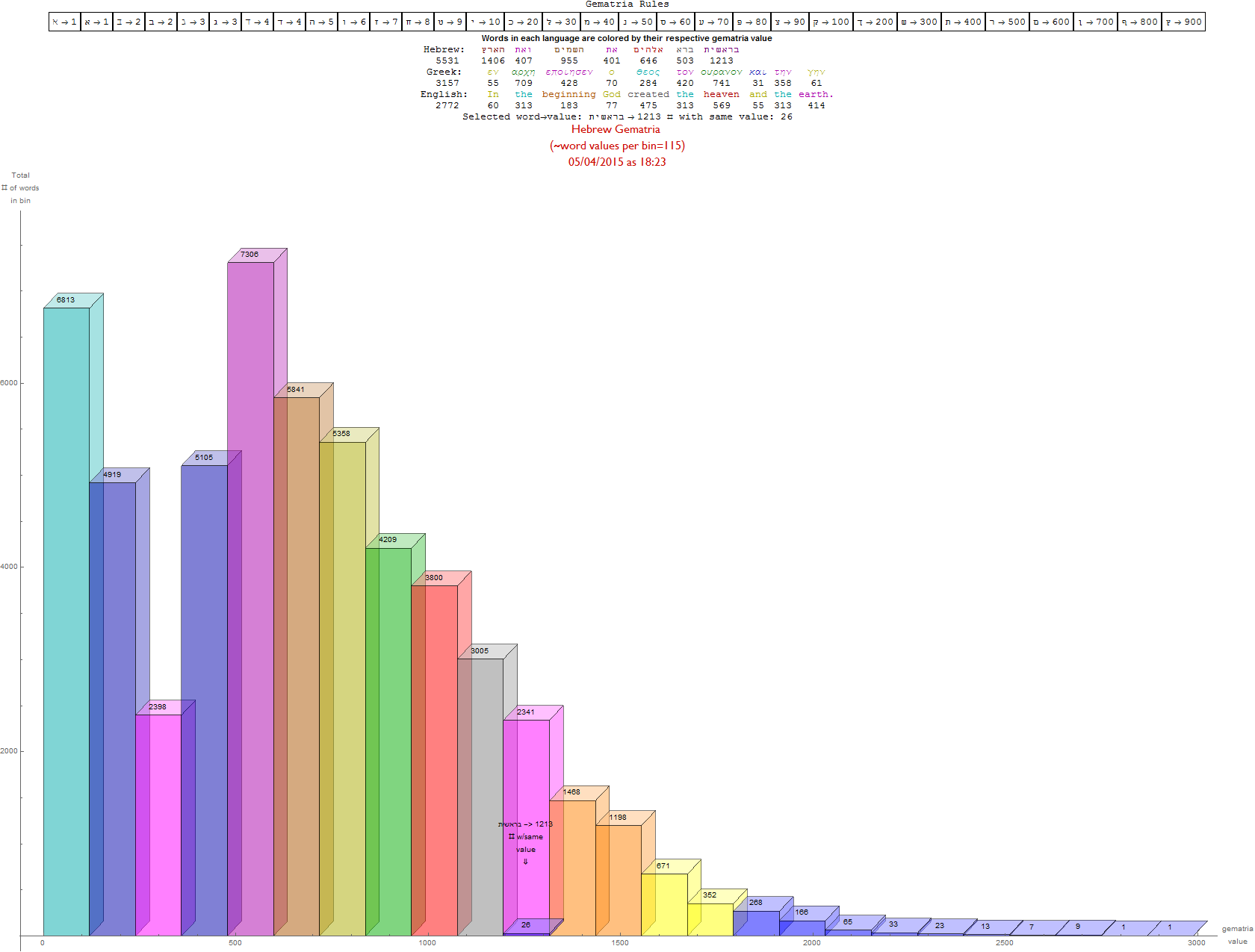The psychology pane deals with the idea of the mathematical beauty of music.

The list of 18 panes is now:
1) Math:____ Chaos/Fibr/Fractal/Surface: Navier Stokes/Hopf/MandelBulb/Klein
2) Math:____ Number Theory:_________ Mod 2-9 Pascal and Sierpinski Triangle
3) Math:____ Geometric Calculus:______ Octonion Fano Plane-Cubic Visualize
4) Math:____ Group Theory:__________ Dynkin Diagram Algebra Create
5) Math:____ Representation Theory:___ E8 Lie Algebra Subgroups Visualize
6) Physics:__ Quantum Elements:______ Fundamental Quantum Element Select
7) Physics:__ Particle Theory:_________ CKM(q)-PMNS(ν) Mix CPT Unitarity
8) Physics:__ Hadronic Elements:______ Composite Quark-Gluon Select Decays
9) Physics:__ Relativistic Cosmology:___ N-Body Bohmian GR-QM Simulation
10) Chemistry: Atomic Elements:_______ 4D Periodic Table Element Select
11) Chemistry:Molecular Crystallography: 4D Molecule Visualization Select
12) Biology:_ Genetic Crystallography:___ 4D Protein/DNA/RNA E8-H4 Folding
13) Biology:_ Human Neurology:________ OrchOR Quantum Consciousness
14) Psychology:Music Theory & Cognition: Chords, Lambdoma, H4, and Tori
15) Sociology: Theological Number Theory: Ancient Sacred Text Gematria
16) CompSci: Quantum Computing:______ Poincare-Bloch Sphere /Qubit Fourier
17) CompSci: Artificial Intelligence:_______ nD Conway’s Game Of Life
18) CompSci: Human/Machine Interfaces:_ nD Human Machine Interface

# The Grand Antiprism

This Grand antiprism was created by projection of 100 of 120 600 cell vertices and 500 edges {488 of 1/2 (3-Sqrt) and 12 of 2/(3+Sqrt)}. One face of this 3D projection contains the VanOss Petrie 30-gon projection. The removed vertices are from the inner rings of the 16-cell and their corresponding 4 Pi/5 rotations in the snub 24-cell.

The other two faces are shown, one of which is seen in the 2D orthonormal projection of H4.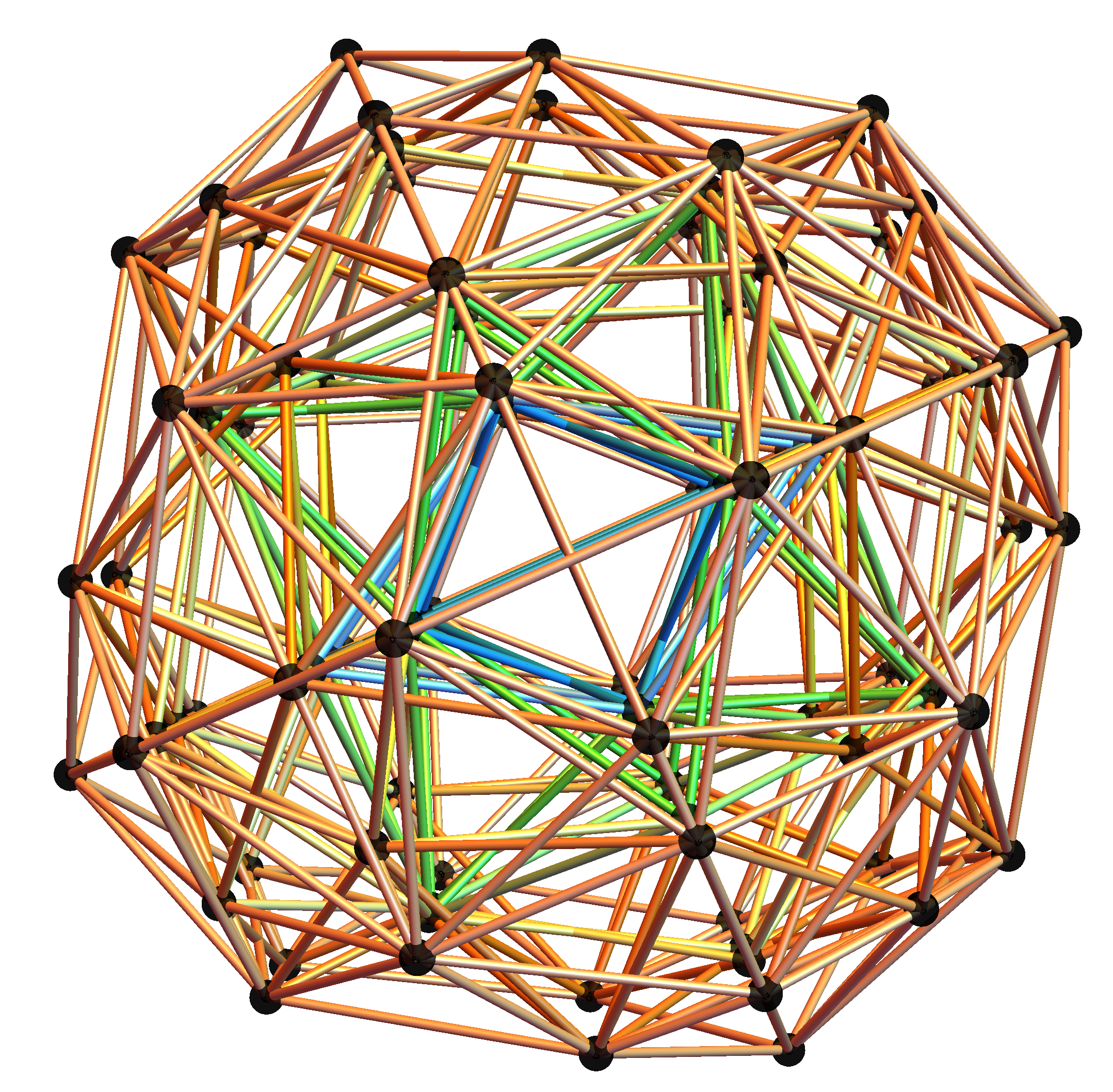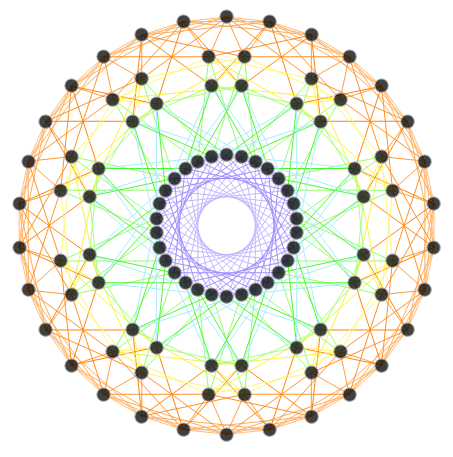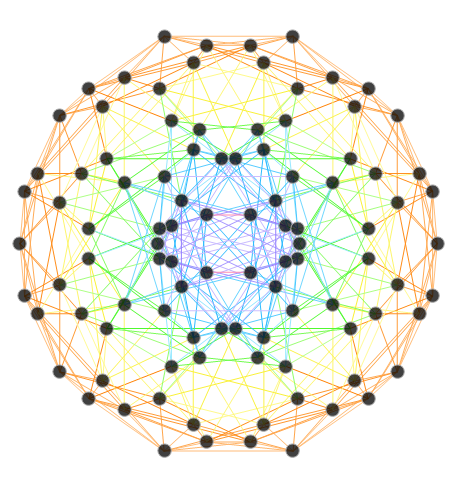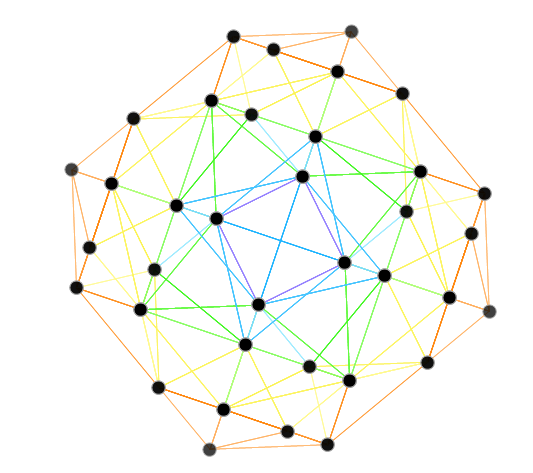# Hopf Fibration and Chaotic Attractors, etc.

I’ve added some new features to my VisibLie_E8 ToE Demonstration. Some of it comes from Richard Hennigan’s Rotating The Hopf Fibration and Enrique Zeleny’s A Collection Of Chaotic Attractors . These are excellent demonstrations that I’ve now included with the features of my integrated ToE demonstration, since they are not only great visualizations, but relate to the high-dimensional physics of E8, octonions and their projections. This gives the opportunity to change the background and color schemes, as well as output 3D models or stereoscopic L/R and red-cyan anaglyph images.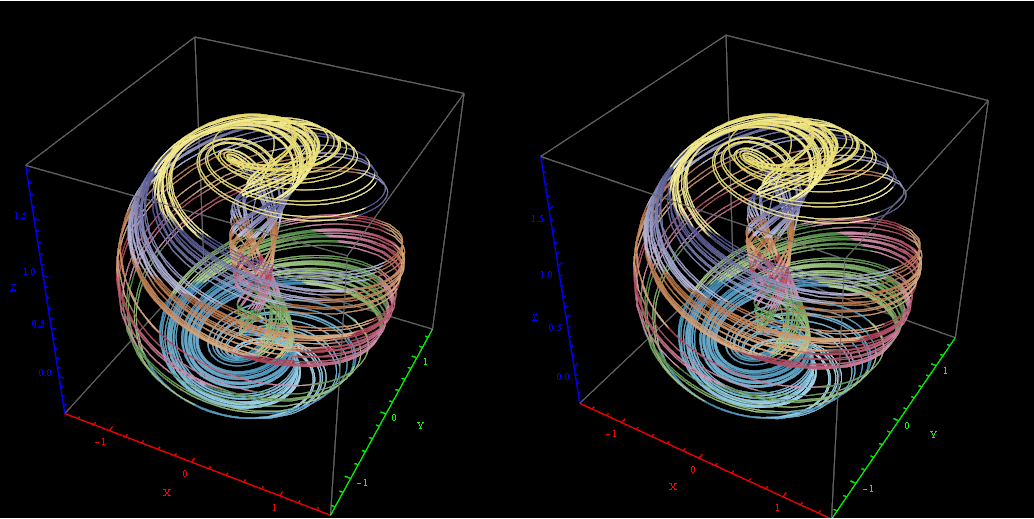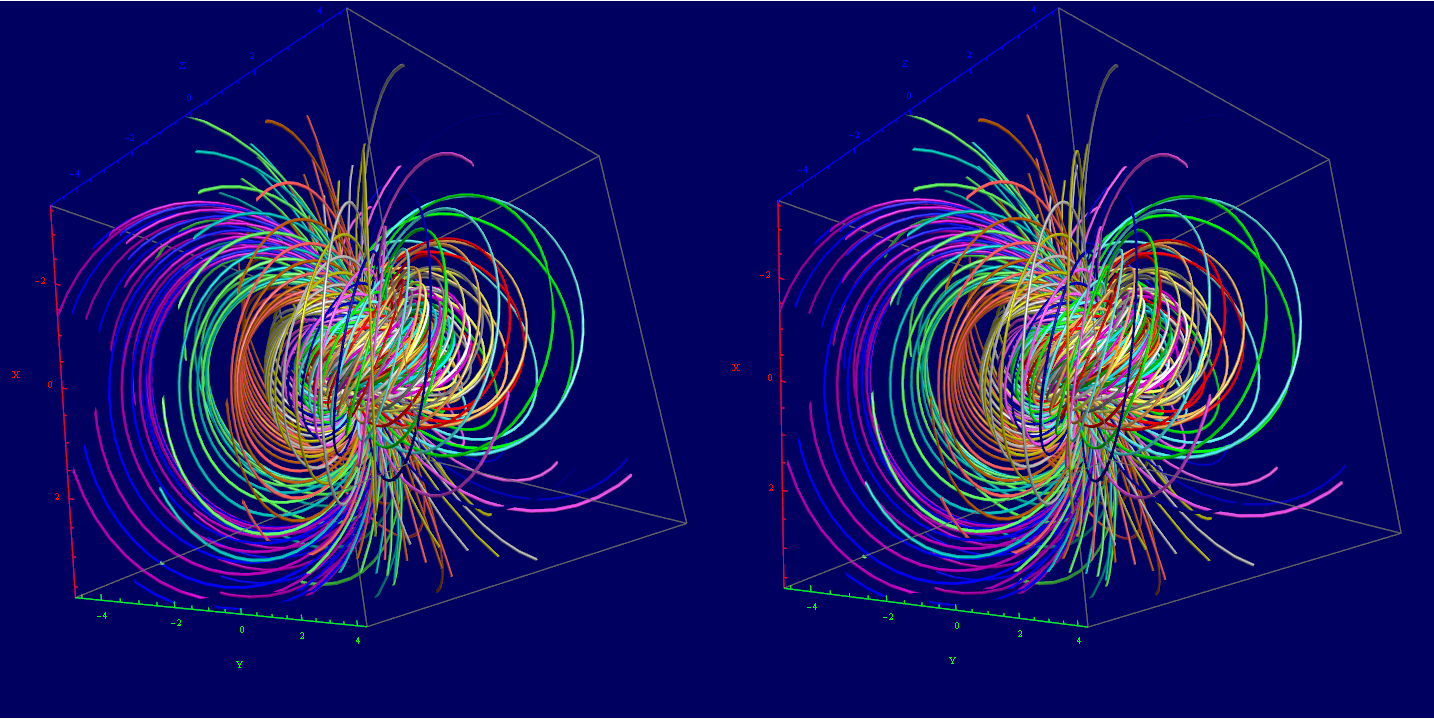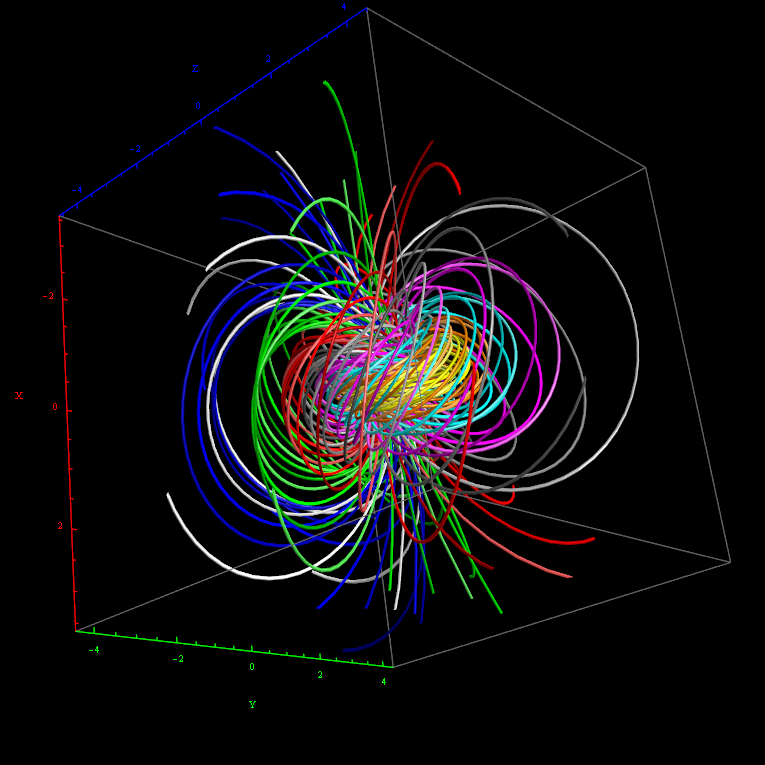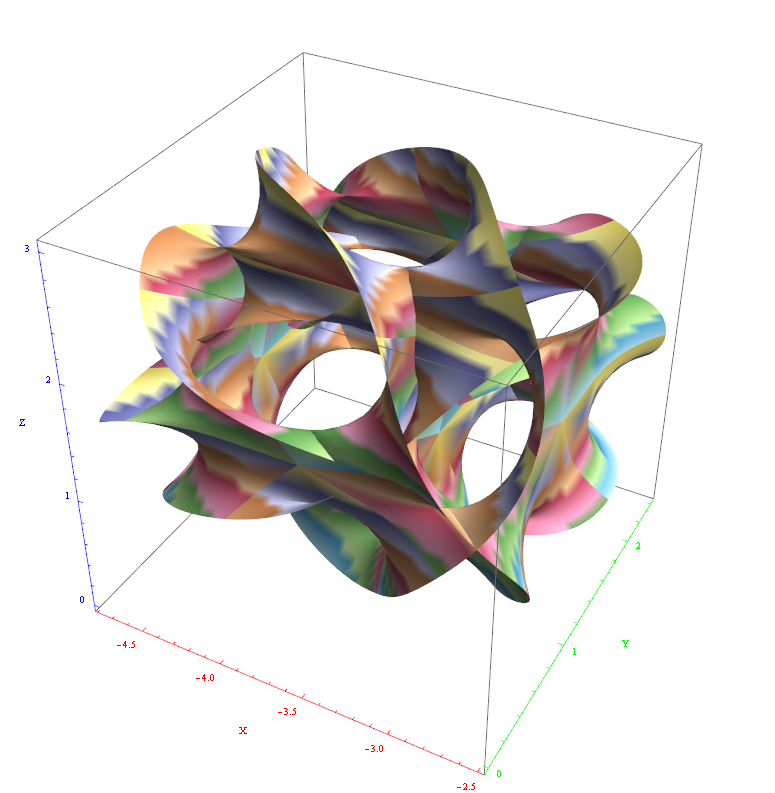I’ve also used David Madore’s help to calculate the symbolic value of the E7 18-gon and 20-gon symmetries of E8. It uses the nth roots of unity (18 and 20, in this case) and applies a recursive dot product matrix based on the Weyl group centralizer elements of a given conjugacy class of E8. I ended up using a combination of Mathematica Group Theory built-in functions, SuperLie and also LieART packages. These symbolic projection values are: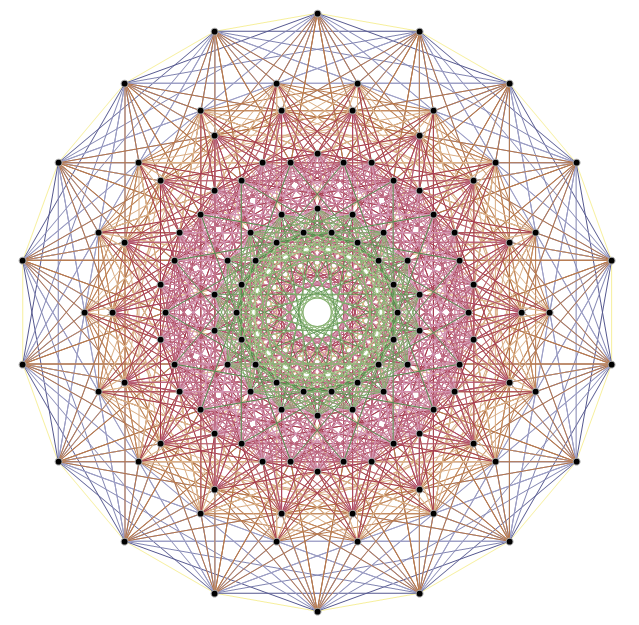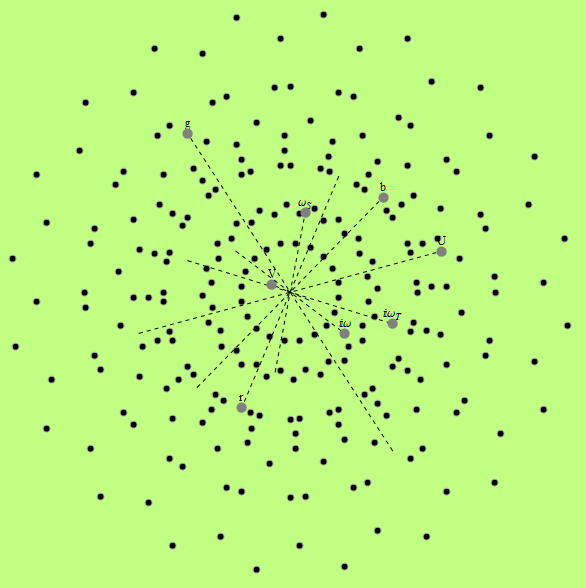# E8 folding to H4+H4/φ

I found the rotation matrix that shows the E8 Dynkin diagram can indeed be folded to H4+H4/φ.

The H4 and its 120 vertices make up the 4D 600 Cell. It is made up of 96 vertices of the Snub 24-Cell and the 24 vertices of the 24-Cell=[16 vertex Tesseract=8-Cell and the 8 vertices of the 4-Orthoplex=16-Cell]).

It can be generated from the 240 split real even E8 vertices using a 4×8 rotation matrix:
x = (1, φ, 0, -1, φ, 0, 0, 0)
y = (φ, 0, 1, φ, 0, -1, 0, 0)
z = (0, 1, φ, 0, -1, φ, 0, 0)
w = (0, 0, 0, 0, 0, 0, φ^2, 1/φ)

where φ=Golden Ratio=(1+Sqrt(5))/2

It is also interesting to note that the x, y, and z vectors project to a hull of the 3D Rhombic Triacontrahedron from the 6D 6 cube Hexaract (which then generates the hull of the Dodecahedron and Icosahedron Platonic solids).

Here’s a look at the Dynkin Diagram folding of E8 to H4+H4/φ: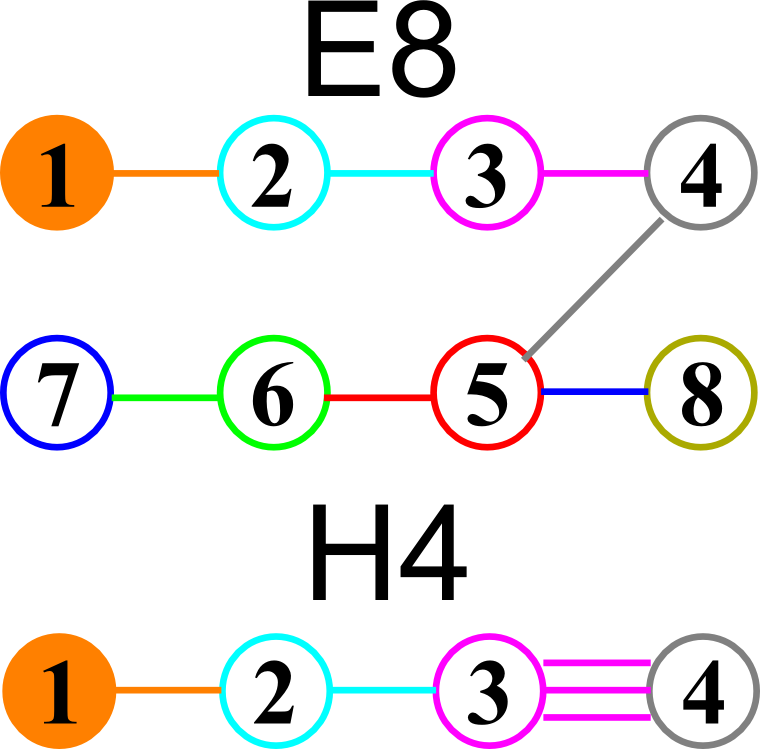I find in folding from 8D to 4D, that the 6720 edge counts split into two sets of 3360 from E8’s 6720 length Sqrt(2), but the combined edges and vertices recreate the E8 petrie diagram perfectly.

Some visualizations of this in 8D: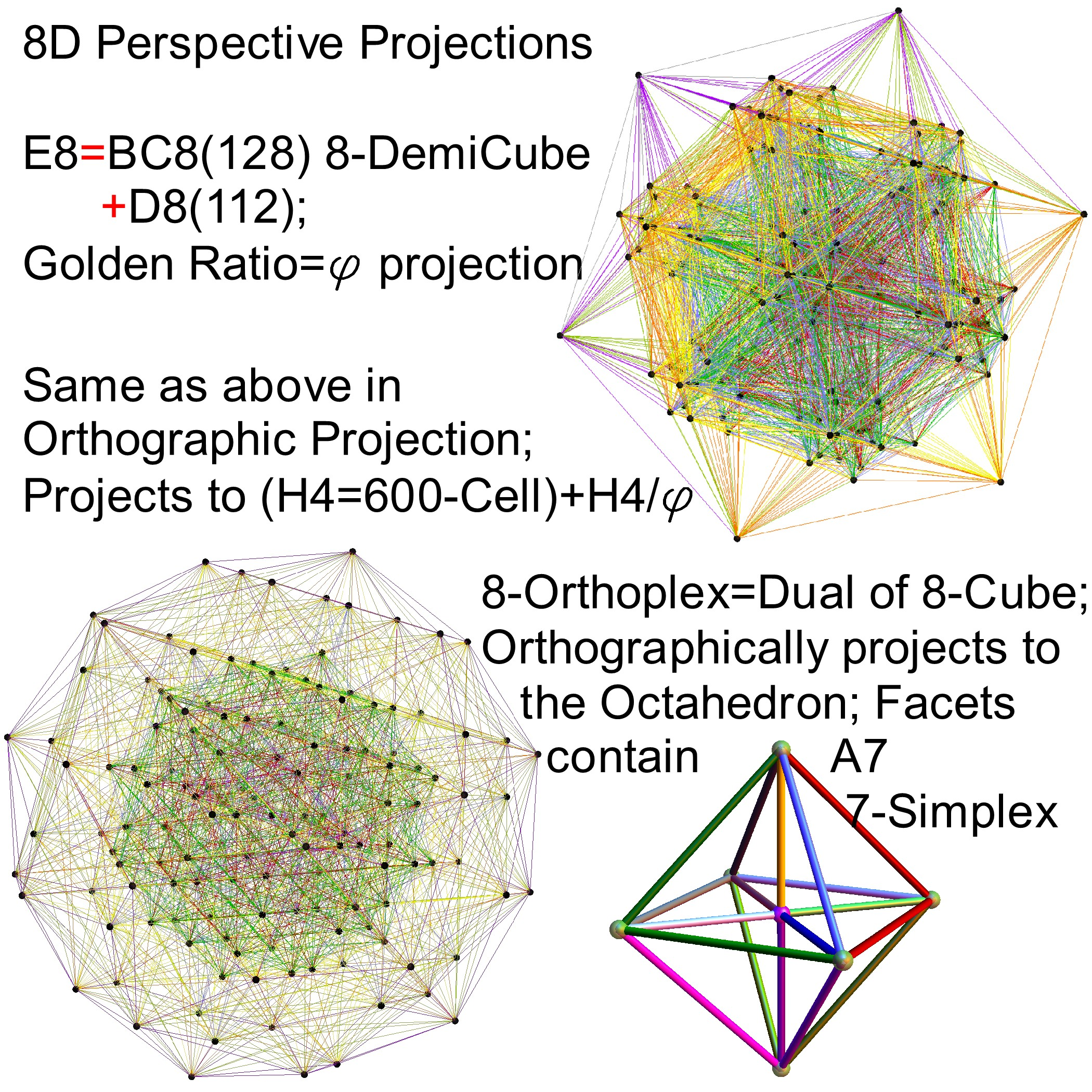and also showing the Rhombic Triacontrahedron folding from 6D: